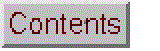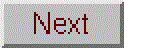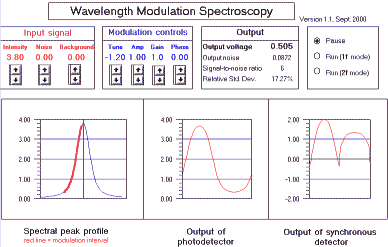Wavelength modulation systemReal-time simulation of a wavelength modulation system, used in some applications of atomic and molecular spectrometry to measure the amplitude of spectral peaks that are superimposed on interfering and unstable background radiation. A wavelength modulation system utilizes a wavelength modulator that varies the wavelength of observation in a periodic manner; it may consist for example of an oscillating voltage applied to a tunable diode laser light source or an oscillating refractor plate installed in the light path inside the entrance slit of a monochromator. When the modulation interval is positioned on a spectral peak, an AC component of the photosignal is generated that is proportional to the peak intensity. The spectral background, on the other hand, typically changes little over the modulation interval and thus produces little or no AC component. A lock-in amplifier is typically used to measure the amplitude of the AC component of the photosignal. The reference signal for the lock-in amplifier is derived from the oscillator that drives the wavelength modulator. (In modern computer- based systems, the functions of the lock-in amplifier may be replaced by the data acquisition software).

Note: these simulations are computationally demanding and run best on 400 MHz Pentium-class machines or better.

Wingz player application and basic set of simulation modules, for windows PCs or Macintosh

Other related simulations:
Lock-in Amplifier
Effect of Slit Width on Emission Spectroscopy SNR

Operating instructions

Click the "Run (1f mode)" radio button (on the right of the screen) to start the real-time simulation. Click "Pause" to freeze the display. The characteristics of the input signal are controlled by the red box labeled "Input signal" on the left; click on the up and down arrows to increase and decrease the intensity of the spectral peak, the random noise, and the background. The peak profile, including noise and background, is shown in the left-hand graph as the blue line (x axis = wavelength; y-axis = intensity).

You can control the wavelength modulation system and the lock-in amplifier with the controls in the middle (blue) box. Tune controls the spectrometer tuning, which positions the wavelength interval relative to the spectral peak. Amp controls the amplitude (wavelength range) of wavelength modulation. As you adjust these controls, you can observe the modulation interval indicated by the red line segment superimposed on the spectral profile in the left-hand graph. The middle graph shows one cycle of the photosignal waveform resulting from wavelength modulation (x axis = time; y-axis = signal voltage); the AC component of this waveform is measured by the lock-in amplifier.

Gain and Phase control the gain and phase of the lock-in amplifier. The right-hand graph shows one cycle of the output of the synchronous detector. (This waveform equals the input signal times gain when the reference signal is positive and equals the negative of the input signal times gain when the reference signal is negative). The output of the lock-in amplifier is shown in the box labeled "Output" on the right. The Output voltage is the DC voltage resulting from low-pass filtering the output of the synchronous detector. The signal-to-noise ratio and the relative standard deviation of the output voltage is also shown here. (The response time of the output voltage is roughly 1 second when the simulation is run on a 450 MHz pentium).

Most real lock-in amplifiers have a second harmonic (2f) mode, in addition to the normal first harmonic (1f) mode. In the 2f mode the reference waveform is frequency- doubled; this is useful when the modulation conditions are such that the photosignal waveform frequency is twice that of the applied wavelength modulation (e.g. when the modulation interval is centered over the spectral peak). In this simulation, the 2f mode is activated by clicking on the "Run (2f mode)" radio button on the upper right.

Mathematical Basis

The spectral peak is modeled as a the sum of Lorentzian
(1/(1+(wavelength)^2)) and Gaussian (exp(-wavelength^2)) components of
equal amplitude, multiplied by the control variable Intensity
(cell \$G\$15). The spectral peak is shown as the thin blue line in
the left-hand graph (x axis = wavelength; y-axis = intensity).

The wavelength (range B19..B208) is modeled as a sine wave whose peak
amplitude is controlled by the "Amp" control (cell \$F\$6). The center of the
modulation interval, relative to the center of the spectral peak, is controlled
by the "Tune" control (cell \$E\$6). The modulation interval is shown as
the thick red line superimposed on the spectral peak in the left-hand graph
(x axis = wavelength; y-axis = intensity).

The signal (range C19..C208) is the result of applying the wavelength
modulation to the spectral peak.

The photomultiplier tube output signal (NoisySignal, range D19..D208) is
calculated by adding signal to random white noise whose amplitude is controlled
by the "Noise" control (cell \$K\$12) and a sloping, fluctuating background whose amplitude
is controlled by the "Background" control (cell \$J\$12). The random white noise has
a maximum frequency that is much higher than the signal frequency (it is sampled
63 times per period of the signal). The background fluctuation has a maximum frequency
that is much lower than the signal frequency; it changes once every four periods
of signal. One cycle of the photodetector output is shown as the red line
in the middle graph (x axis = time; y-axis = signal voltage or current).

The reference waveform (range F19..F208) is a sine wave. There are two modes of
operation, selected by the radio buttons on the top right of the screen. In
the 1f mode, the frequency of the reference waveform is equal to that
of the wavelength modulation. In the 2f mode, the frequency of the reference
waveform is twice that of the wavelength modulation. The equation
for the reference waveform ((sin(phase+time)^Frequency)-Offset) makes
use of the property that the square of a sine function is a sine function of
double the frequency. In either mode the reference waveform is phase-shifted
by an amount controlled by the "phase" control (cell \$D\$18).

The synchronous detector output (range H19..H208) is given by the product of the
gain, the photomultiplier tube output signal (NoisySignal), and the sign of the
reference waveform; that is, when the reference waveform is positive, NoisySignal
is passed unchanged; when the reference waveform is negative, NoisySignal is
inverted. One cycle of the synchronous detector output is displayed as the red
line in the right-hand graph (x axis = time; y-axis = signal voltage). Because the
purpose of the lock-in amplifier is to measure the AC component of the input signal,
the DC component of the signal is eliminated by subtracting the Average value
of NoisySignal (cell \$H\$18) from itself before computing the synchronous detector
output.

In an analog lock-in amplifier, the synchronous detector output is fed through
a low-pass filter before being displayed. To simulate this effect,
the average of the synchronous detector output over a period of 60 cycles is
computed and displayed as the "Output Voltage" (cell K5). The standard deviation
of the output voltage is estimated and displayed as "Output noise" (cell K6),
along with the signal-to-noise ratio (output voltage divided by standard deviation)
and the relative standard deviation (standard deviation divided by output voltage).

Variables: Intensity = \$G\$15 (peak intensity of the spectral line or band)
noise = \$K\$12 (peak amplitude of the random noise)
BackgroundAmp = \$J\$12 (peak amplitude of the background)
Tune = \$E\$6 (wavelength of the modulation interval relative to spectral peak)
Amp = \$F\$6 (amplitude of the wavelength modulation interval)
Gain = \$G\$6 (gain of the lock-in amplifier)
phase = \$F\$18 (phase of the reference waveform relative to the wavelength modulation)
background = \$D\$18 (background added to signal waveform)=BackgroundAmp*rand()/40+1
Frequency= \$E\$18 (Frequency multiplier; equals 1 in 1F mode and 2 in 2F mode)
Offset = \$G\$18 (reference offset for 2F mode; equals 0 in 1F mode and 0.5 in 2F mode)
Average = \$H\$18 (=avg(C19..C82)) average DC level of the detector output

Array calculations:
A19..A208: time (0 to 18.9 in steps of 0.1)
B19..B208: wavelength = Amp*sin(time)+Tune
C19..C208: signal = Intensity * ((1/(1+(wavelength)^2))+exp(-wavelength^2))
+ BackgroundAmp*(wavelength+5)/20*background
D19..D208: NoisySignal = signal + background+noise*(rand()-rand())
F19..F208: reference = (sin(phase+time)^Frequency)-Offset
H19..H208: detector output = Gain*sign(reference)*(NoisySignal-Average)
A note about the use of the rand() function: The rand()-rand() formulation
seen in the above equations is used to produce a random variable with a mean of
zero and a more nearly normal distribution. By itself, the rand() function
returns a random number that is uniformly distributed between 0 and 1.

Suggested experiment

Simulation of a wavelength modulation system

1. Start with Intensity set to maximum (3.80), Noise = 0, Background = 0, Gain = 1. and Phase = 0. Click the "Run (1f mode)" radio button to start the real-time simulation.

2. Vary the Tune and Amp variables by clicking on the up and down arrows under the numbers and observe the effect on the graphs. Tune controls the spectrometer tuning, which positions the wavelength interval relative to the spectral peak. Amp controls the amplitude (wavelength range) of wavelength modulation. As you adjust these controls, observe the modulation interval indicated by the red line segment superimposed on the spectral profile in the left-hand graph. The photosignal waveform resulting from the wavelength modulation is shown in the middle graph. Note how this waveform changes as you vary Tune and Amp.

3. Set Amp = 0.25 and Tune = -1, so that the modulation interval covers a small region on the sloping side of the spectral peak where the slope is positive and where the spectral profile is roughly a straight line. In this case the resulting photosignal waveform (output of the photomultiplier tube) is a sine wave, because the wavelength modulation waveform itself is a sine wave. Note that the waveform goes up (in the positive direction) in the first half of the waveform and moves down (in the negative direction) in the second half of the waveform, just like a "textbook" sine wave. That's because the slope of the spectral profile within the modulation interval is positive.

4. Now look at the output of the synchronous detector shown in the right- hand graph. Note that in the first half of the waveform, the photosignal waveform is passed without change, forming a positive bump, whereas in the second half of the waveform, the photosignal waveform is inverted, forming a second positive bump. When this waveform is smoothed by the output low-pass filter, its amplitude (displayed as the "Output voltage") is a positive voltage (it should read 0.371 volts). While you're at it, vary the Phase control. This control changes the phase angle between the reference waveform and the wavelength modulation; that is, it determines where in the output cycle the waveform is inverted and where it is left alone. As you vary Phase, you can observe the changes in the output of the synchronous detector and in the Output voltage. Phase should be adjusted to give the largest Output voltage; the optimum setting is 0.0 in this simulation.

5. Now move the modulation interval to the other side of the peak by setting Tune to 1.0. Now the photosignal waveform goes down in the first half of the waveform and moves up in the second half of the waveform, just the opposite of before. Note the effect on the synchronous detector output shown in the right-hand graph. In the first half of the waveform, the photosignal waveform is passed without change as before, forming in this case a negative bump, whereas in the second half of the waveform, the photosignal waveform is inverted, forming a second negative bump. When this waveform is smoothed by the output low-pass filter, its amplitude (displayed as the "Output voltage" is a negative voltage (it should read -0.371 volts).

In this simulation, the spectral peak is perfectly symmetrical, so either side of the peak can be used equally well. It makes little practical difference whether the output voltage is actually positive or negative. In practical spectroscopic applications, spectral interferences that fall on or near one side of a peak may make that side less usable.

6. Increase the value of Amp and note that the amplitude of the photosignal modulation increases. At large values of Amp, the photosignal becomes "distorted" and does not look much like a sine wave, but nevertheless the Output voltage continues to increase. What is the largest output voltage you can get by varying the values of both of Tune and Amp? You may be surprised by how "strange" the photosignal waveform appears under conditions that give the largest output voltage.

7. So far, all the experiments have been with the noise set to zero, in order to make the shape of the waveforms easier to observe clearly. However, in real practical applications, the input signal always contains random noise and other disturbances. In fact, in many cases the noise is larger than the signal itself.

Leave the Intensity to maximum and increase Noise to 0.08. This adds a small amount or random white noise to the input signal. Note that this noise is evident in the graph of the input signal, the photodetector signal, and in the synchronous detector output. The result of this noise is that the Output voltage is no longer steady but rather fluctuates slightly around its mean value. The Output noise readout reports a reading of about 0.0011, which is obviously much lower than the input noise of 0.08. As a result, the signal-to-noise ratio (SNR) has been improved as well; the input SNR = 3.8/0.08 = 47.5, whereas the output SNR is approximately 900. This noise reduction and SNR improvement is due to the output low-pass filtering on the lock-in amplifier. If you increase the noise setting, the output noise will increase proportionally, the output SNR will decrease proportionally, and the displayed Output voltage will become less and less steady, although always fluctuating around its correct value.

What is the largest noise setting that will still allow you to distinguish reliably between an Intensity of 0.0 and 0.2, as judged by inspecting the Output voltage?

8. Now return Noise to zero and increase the Background. This superimposes the spectral profile on a sloping background, simulating a commonly encountered type of spectroscopic interference. You will also notice that his background is slightly unstable, and that the background fluctuation occurs at frequencies below the wavelength modulation frequency (in contrast to the random noise, which occurs at higher frequencies). As you increase the Background, you can easily see the effect that it has on the photodetector output. and on the synchronous detector output: it increases the magnitude of the AC signal at the modulation frequency and thus increases the output voltage, even though the actual intensity of the spectral peak has not really increased. You can set the peak Intensity to zero and still there is a small output voltage due to the background. The reason for this situation is that the background is sloping over the modulation interval, and thus it generates an AC signal much like the actual spectral peak. To get a true reading of the Output voltage, you have to subtract out the reading you get with the peak Intensity set to zero. However, in a real application, this could lead to measurement errors if the background were to change during a measurement.

9. The solution to the background problem is to operate in the so-called "2f mode". In this mode the modulation interval is positioned across the center of the spectral peak so that it extends over both sides of the peak. To demonstrate this mode, temporarily set Noise and Background to zero; then set Tune to zero. Notice that the photodetector output now shows two cycles, indicating that the frequency of the AC signal has been doubled (hence the name "2f"). In order for the lock-in amplifier to detect this frequency, its reference frequency must also be doubled by switching it to the 2f mode; in the simulation this is done by clicking on the "2f mode" radio button in the upper right. The Output voltage should now show a voltage that is proportional to the spectral peak intensity. (However, a slight inconvenience is that the Output voltage is now negative, due to the phase of the signal. This is not a serious problem, but it can be corrected if desired by setting the Phase control to 1.60 while in the 2f mode).

In the 2f mode, the lock-in amplifier is quite effective in reducing the effect of the background and its fluctuation. Even if the Background is higher that the spectral peak itself, the output voltage is quite steady. You can easily demonstrate that an equivalent amount of random noise causes much more variation in the output voltage: compare the effect of a Noise = 0.08 to the effect of Background = 2.00; the amplitude of the fluctuations are about the same, but the output noise produced by Background = 2.00 is much less that that produced by Noise = 0.08. This is because most of the background fluctuation falls at frequencies below the modulation frequency, whereas the random noise is "white" and occurs at all frequencies.

Compare the efficiency of the 1f and 2f modes at reducing background by setting Intensity to 0.20, Noise to 0.0, and Background to 1.00. What is the best SNR you can get in both modes by varying Tune and Amp?

(c) 1991, 2015. This page is part of Interactive Computer Models for Analytical Chemistry Instruction, created and maintained by Prof. Tom O'Haver , Professor Emeritus, The University of Maryland at College Park. Comments, suggestions and questions should be directed to Prof. O'Haver at toh@umd.edu. Number of unique visits since May 17, 2008: